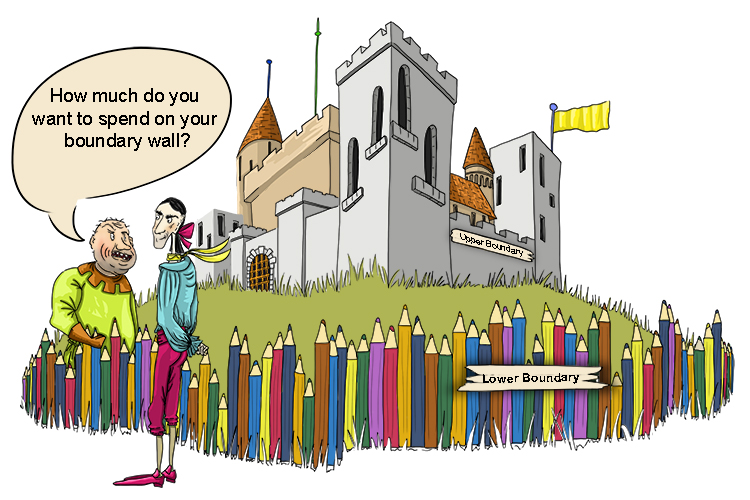# Upper and lower bound – Boundary possible values

If a number has been rounded it is important to realise what possible values the exact value might have been.You want a boundary (bound) wall – What are your limits?

Upper boundary – The largest value that a number can be (in this boundary).

Lower boundary – The smallest value that a number can be (in this boundary).

Remember:

Underline the digit
Look next door
Five or more raise the score
Four or less just ignore

Example 1

What are the upper and lower bounds of the following number, which has been rounded to one decimal place? 743.8

Use the following process to solve:

743.8                 To one decimal place

743.8                 Underline the digit (in this case 8)

743.80               Look next door

743.80 and lower boundary - Five or more, would raise the score

If it was 743.75 the "Five or more, raise the score" would still raise this to 743.8 so the lower boundary is 743.75.

743.80 and upper boundary - Four or less, just ignore

If it was 743.84999 the "Four or less, just ignore" would reduce this to 743.8 so the upper boundary is 743.84999, but unbelievably mathematicians still call this 743.85 to simplify the answer.

Answer: The upper and lower boundaries are 743.85 and 743.75 respectively.

Notice this could have been achieved simply by adding and subtracting 0.05.

So 743.8 upper and lower boundary is

743.8+0.05=743.85 Upper boundary

743.8-0.05=743.75 Lower boundary

Example 2

53 mph has been rounded to the nearest mile per hour. What are the upper and lower boundaries?

53                       Rounded to the nearest mile per hour

53                       Underline the digit (in this case 3)

53.0                    Look next door

53.0 and lower boundary - Five or more, would raise the score

If it were 52.5 the "Five or more, raise the score" would still raise this to 53.0 so the lower boundary is 52.5.

53.0 and upper boundary - Four or less, just ignore

If it were 53.4999 the "Four or less, just ignore" would reduce this to 53.0 so the upper boundary is 53.4999, but unbelievably mathematicians still call this 53.5 to simplify the answer.

Answer: The upper and lower boundaries are 53.5 and 52.5 respectively.

Notice this could have been achieved simply by adding and subtracting 0.05.

So 53 upper and lower boundary is

53+0.5=53.5 Upper boundary

53-0.5=52.5 Lower boundary

Example 3

5300 has been rounded to two significant figures. What are the upper and lower boundaries?

5300                   Rounded to two significant figures

5300                   Underline the digit (in this case 3)

5300                   Look next door

5300+50=5350 Upper boundary

5300-50=5250 Lower boundary

Answer: The upper and lower boundaries are 5350 and 5250 respectively.